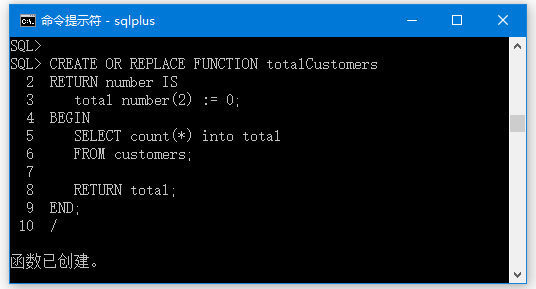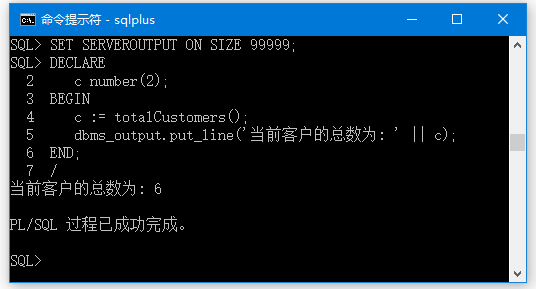## 1. 创建函数

CREATE [OR REPLACE] FUNCTION function_name
[(parameter_name [IN | OUT | IN OUT] type [, ...])]
RETURN return_datatype
{IS | AS}
BEGIN
< function_body >
END [function_name];

• function-name是指定要创建的函数的名称。
• [OR REPLACE] 选项指示是否允许修改现有的函数。
• 可选参数列表包含参数的名称，模式和类型。 IN表示将从外部传递的值，OUT表示将用于返回过程外的值的参数。
• 函数必须包含一个返回(RETURN)语句。
• RETURN子句指定要从函数返回的数据类型。
• function-body包含可执行部分。
• 使用AS关键字代替IS关键字，用来创建独立的函数。

### 示例

CREATE TABLE CUSTOMERS(
ID   INT NOT NULL,
NAME VARCHAR (20) NOT NULL,
AGE INT NOT NULL,
SALARY   DECIMAL (18, 2),
PRIMARY KEY (ID)
);

-- 数据
VALUES (1, 'Ramesh', 32, 'Ahmedabad', 2000.00 );

VALUES (2, 'Khilan', 25, 'Delhi', 1500.00 );

VALUES (3, 'kaushik', 23, 'Kota', 2000.00 );

VALUES (4, 'Chaitali', 25, 'Mumbai', 6500.00 );

VALUES (5, 'Hardik', 27, 'Bhopal', 8500.00 );

VALUES (6, 'Komal', 22, 'MP', 4500.00 );

CREATE OR REPLACE FUNCTION totalCustomers
RETURN number IS
total number(2) := 0;
BEGIN
SELECT count(*) into total
FROM customers;

END;
/## 2. 调用函数

SET SERVEROUTPUT ON SIZE 99999;
DECLARE
c number(2);
BEGIN
c := totalCustomers();
dbms_output.put_line('当前客户的总数为: ' || c);
END;
/### 示例

SET SERVEROUTPUT ON SIZE 99999;
CREATE OR REPLACE FUNCTION findMax(x IN number, y IN number)
RETURN number
IS
z number;
BEGIN
IF x > y THEN
z:= x;
ELSE
Z:= y;
END IF;
RETURN z;
END;
/
DECLARE
a number;
b number;
c number;
BEGIN
a:= 23;
b:= 45;
c := findMax(a, b);
dbms_output.put_line('两个数：23,45 之中的最大值是: ' || c);
END;
/

两个数：23,45 之中的最大值是: 45

## 3. PL/SQL递归函数

n! = n*(n-1)!
= n*(n-1)*(n-2)!
...
= n*(n-1)*(n-2)*(n-3)... 1

SET SERVEROUTPUT ON SIZE 99999;
DECLARE
num number;
factorial number;

FUNCTION fact(x number)
RETURN number
IS
f number;
BEGIN
IF x=0 THEN
f := 1;
ELSE
f := x * fact(x-1);
END IF;
RETURN f;
END;

BEGIN
num:= 10;
factorial := fact(num);
dbms_output.put_line(' 数字 '|| num || ' 的阶乘积是： ' || factorial);
END;
/

数字 10 的阶乘积是： 3628800

PL/SQL 过程已成功完成。